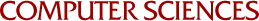On Multivariate Approximation

Integer Translates of a Basic Function by Nira Dyn, IRH Jackson, D Levin and Amos Ron
1989

Approximation properties of the dilations of the integer translates of a smooth function, with some derivatives vanishing at infinity, are studied. The results apply to fundamental solutions of homogeneous elliptic operators and to shifted fundamental solutions of the iterated Laplacian. Following the approach from spline theory, the question of polynomial reproduction by quasi-interpolation is addressed first. The analysis makes an essential use of the structure of the generalized Fourier transform of the basis function. In contrast with spline theory, polynomial reproduction is not sufficient for the derivation of exact order of convergence by dilated quasi-interpolants. These convergence orders are established by a careful and quite involved examination of the decay rates of the basis function. Furthermore, it is shown that the same approximation orders are obtained with quasi-interpolants defined on a bounded domain.